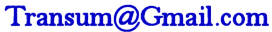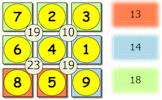## An exercise on rounding values in a calculation to find an approximate estimate of the answer.

##### RoundingLevel 1Level 2Level 3Level 4BoundsExam-StyleDescriptionHelpMore ...

This is level 4: real life problems and puzzles. You will be awarded a trophy if you get at least 9 correct and you do this activity online.

 Andrew drinks 1.9 litres of water each day for three years. By rounding the amount of water an the number of days to one significant figure find the approximate amount of water he drinks during the three years. [One year = 365 or 366 days] Working: $$l$$Bella estimates the number of pounds in 895 kilograms using the fact that $$1kg$$ ≈ $$2.2lb$$ and by rounding the numbers she uses in her calculation to one significan figure. What was the answer she got? Working: $$lb$$Chris makes a quick estimate of how many people weighing $$82kg$$ will fit into a lift with a maximum load warning of $$910kg$$. He does this by rounding the values used in the calculation to one significant figure. What was his answer? Working:Write down this calculation with each of the numbers rounded to one significant figure. Now work out the value of this new calculation.$$\frac{99.2 + 81}{4.27 - 22.9 ÷ 11.5}$$ Working:Diana changed £915 to Fijian Dollars($) when the rate was £1 =$2.863. She first estimated the amount she would receive by rounding the two numbers she used in her calculation to one significant figure. How many dollars did she expect to receive? Working: \$What is the value of the next square number after $$2.55^2$$? Working:What is the value of the next cube number after $$5.18^3$$? Working:Calculate an estimate for the circumference of a circle with diameter 7.88cm by rounding the numbers used in your calculation to one significant figure. Working: cmThe average amount of storage space needed for a photograph taken with Ed's phone is 3940 KB. The available storage space on Ed's laptop is 217 GB. By rounding these numbers to one significant figure estimate the number of photographs that could be stored on Ed's laptop. Working:Write down this calculation with each of the numbers rounded to the nearest integer. Now work out the value of this new calculation giving your answer to the nearest integer.$$\sqrt{(6.4+18.5)(15.8-13.6)}$$ Working:Write down this calculation with each of the numbers rounded to one significant figure. Now work out the value of this new calculation.$$\frac{0.000189 \times 1300}{\sqrt{3760 \div 36.7}}$$ Working:It is said that in an average lifetime of 79 years, each human is likely to swallow about 9 spiders while sleeping. Supposing that the population of the UK is around 67 million, estimate of the number of spiders consumed in this way in the UK each year? Give your answer correct to one significant figure. Working:Check

This is Rough Answers level 4. You can also try:
Level 1 Level 2 Level 3

## Instructions

Try your best to answer the questions above. Type your answers into the boxes provided leaving no spaces. As you work through the exercise regularly click the "check" button. If you have any wrong answers, do your best to do corrections but if there is anything you don't understand, please ask your teacher for help.

When you have got all of the questions correct you may want to print out this page and paste it into your exercise book. If you keep your work in an ePortfolio you could take a screen shot of your answers and paste that into your Maths file.

## Transum.org

This web site contains over a thousand free mathematical activities for teachers and pupils. Click here to go to the main page which links to all of the resources available.## More Activities:

Mathematicians are not the people who find Maths easy; they are the people who enjoy how mystifying, puzzling and hard it is. Are you a mathematician?

Comment recorded on the 9 October 'Starter of the Day' page by Mr Jones, Wales:

"I think that having a starter of the day helps improve maths in general. My pupils say they love them!!!"

Comment recorded on the 7 December 'Starter of the Day' page by Cathryn Aldridge, Pells Primary:

"I use Starter of the Day as a registration and warm-up activity for my Year 6 class. The range of questioning provided is excellent as are some of the images.
I rate this site as a 5!"

#### Suko SujikoInteractive number-based logic puzzles similar to those featuring in daily newspapers designed to develop numeracy skills. These puzzles are drag and drop and can earn you a Transum Trophy.

There are answers to this exercise but they are available in this space to teachers, tutors and parents who have logged in to their Transum subscription on this computer.

A Transum subscription unlocks the answers to the online exercises, quizzes and puzzles. It also provides the teacher with access to quality external links on each of the Transum Topic pages and the facility to add to the collection themselves.

Subscribers can manage class lists, lesson plans and assessment data in the Class Admin application and have access to reports of the Transum Trophies earned by class members.

Subscribe

## Go Maths

Learning and understanding Mathematics, at every level, requires learner engagement. Mathematics is not a spectator sport. Sometimes traditional teaching fails to actively involve students. One way to address the problem is through the use of interactive activities and this web site provides many of those. The Go Maths page is an alphabetical list of free activities designed for students in Secondary/High school.

## Maths Map

Are you looking for something specific? An exercise to supplement the topic you are studying at school at the moment perhaps. Navigate using our Maths Map to find exercises, puzzles and Maths lesson starters grouped by topic.

## Teachers

If you found this activity useful don't forget to record it in your scheme of work or learning management system. The short URL, ready to be copied and pasted, is as follows:

Jen, Australia

Saturday, June 1, 2019

"I just tried level one. It looks like a great way to reinforce significant figures to students, through mental estimates! However, the question says give answers to one significant figure - and I just got most of the questions "wrong", as your answers are mostly to 2 significant figures."

Transum,

Sunday, June 2, 2019

"Thanks very much for your feedback Jen. The instructions ask for the numbers in the questions to be rounded to one significant figure; not the answers. The answers should be given exact after the pre-calculation rounding has taken place. Once again thanks for the feedback, much appreciated."

Do you have any comments? It is always useful to receive feedback and helps make this free resource even more useful for those learning Mathematics anywhere in the world. Click here to enter your comments.For All:

© Transum Mathematics :: This activity can be found online at:
www.transum.org/Maths/Exercise/Approximating/Default.asp?Level=4

## Description of Levels

CloseRounding - Before doing these exercises you will need to know how to round numbers to one significant figure.

Level 1 - Round the numbers in a basic calculation to one significant figure to obtain an approximate answer

Level 2 - Round the numbers to one significant figure to obtain an approximate answer to calculations including decimals and fractions

Level 3 - Determine whether an approximation is a over-estimate or an under-estimate if each of the numbers are rounded to one significant figure

Level 4 - Real life problems and puzzles

Bounds - Determine the upper and lower bounds when rounding or truncating quantities used in calculations.

Exam Style Questions - A collection of problems in the style of GCSE or IB/A-level exam paper questions (worked solutions are available for Transum subscribers).

Breathe Easily - A real-life question that can be (roughly) answered by making some approximations.

Fermi Problem - Unleash your approximating skills to estimate something quite unknown in this Advanced Starter.

More on this topic including lesson Starters, visual aids, investigations and self-marking exercises.

Answers to this exercise are available lower down this page when you are logged in to your Transum account. If you don’t yet have a Transum subscription one can be very quickly set up if you are a teacher, tutor or parent.

## Curriculum Reference

See the National Curriculum page for links to related online activities and resources.

## Example

The video above is from the maths520 channel.

Don't wait until you have finished the exercise before you click on the 'Check' button. Click it often as you work through the questions to see if you are answering them correctly. You can double-click the 'Check' button to make it float at the bottom of your screen.

Answers to this exercise are available lower down this page when you are logged in to your Transum account. If you don’t yet have a Transum subscription one can be very quickly set up if you are a teacher, tutor or parent.

Close